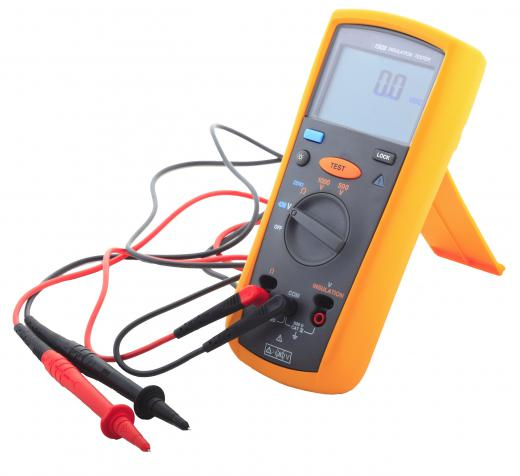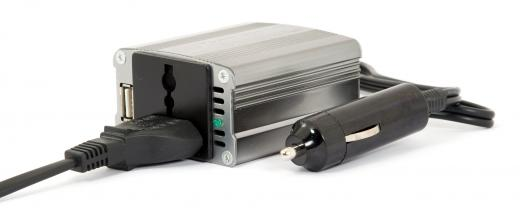# What is Impedance?

S. Mithra
S. MithraA multimeter can be used to measure impedance.

In electrical engineering, impedance is a measure of the extent to which a circuit opposes the flow of electricity. All materials have some degree of electrical resistance, which causes some energy to be lost as heat, and reduces the flow of current. In the case of direct current (DC), impedance is the same as resistance, and depends solely upon the materials from which the circuit is made. For an alternating current (AC), however, two additional factors can contribute to the impedance: capacitance and inductance. Together these are known as reactance, which is a measure of opposition to a change in current that depends on its frequency, and on the components of the circuit.A power inverter, which can be used to convert DC to AC.

Alternating current is constantly changing direction, and does so at a given frequency, which is measured in Hertz (Hz), or cycles per second. Typically, electricity is supplied at 50 or 60 Hz, but this may be changed for specific applications. The frequency can be displayed as a wave on an oscilloscope in terms of current or voltage, with the distance from crest to crest representing a complete cycle. The degree of reactance in a circuit is dependent upon the frequency of the AC supply. More specifically, capacitive reactance decreases with increasing frequency, while inductive reactance increases.

#### Capacitive Reactance

A capacitor is a device that can store an electrical charge, and later release it. It generally consists of a non-conducting material, or insulator, sandwiched between two metal plates. As part of a circuit, it allows a charge to build up in the insulator and effectively stores energy in an electric field. As the charge increases, the current is reduced. After a certain time, the capacitor will be unable to absorb any more charge and the current will drop to zero, at which point it will discharge, producing a flow of electrons in the opposite direction.

If, however, the AC frequency is high, the current will change direction in less time than the capacitor takes to “fill up.” Since the current is at its maximum at the start of a cycle, a high frequency AC supply will be virtually unaffected by a capacitor. In contrast, if the frequency is low, this will allow time for some charge to build up in the capacitor, causing a reduction in current before the next cycle. Capacitors are used in many popular devices and gadgets, and so capacitive reactance is usually an important factor in impedance.

#### Inductive Reactance

Inductance is the tendency of a changing current flowing through a wire to induce an opposing current in a nearby conductor. This happens because a changing electrical current produces a changing magnetic field, which in turn causes electrons to flow in any conducting material within its range. When a wire is wound into a coil, it forms an inductor, and will induce an opposing flow of electrons, or electromotive force (EMF) in itself. The voltage of the induced EMF increases with the rate of change of the supply voltage, so increasing the AC frequency will increase the inductive reactance. Like capacitors, inductors are commonly used components.

#### Capacitors and Inductors in Combination

When both devices are present in a circuit, the effects depend not only on the AC frequency, but also on how they are wired. If a capacitor and inductor are connected in series, the current initially rises with frequency, reaching a maximum at a certain point, known as the resonance frequency, and falls thereafter. If they are wired in parallel, the current falls with rising frequency until a point is reached where none flows. Beyond this point, the flow rises again.

#### Measurement and Units

Like resistance, reactance and impedance are measured in ohms. In equations, impedance is normally represented by the symbol Z, and reactance by X. Capacitive and inductive reactance are represented by XC and XL, respectively. Similarly to Ohm’s law for resistance, overall impedance can be expressed as Z=V/I, where Z is given in ohms; V is voltage, given in volts; and I is current, given in amps.anon128541

Impedance is Z

Reactance is X

Resistance is R

Z{squared} = R{squared} + X{squared}

anon90308

Is impedance mhz or is it the tip of the radio frequency that touches the skin?

anon76390

How it is calculated?

anon60307

what is the formula to calculate ohmic value for transformer impedance?

anon58600

can you please give me the full concept of impedance?

anon52638

Please explain about impedance used in complex numbers. thank you.

anon49679

why is transformer secondary always designed for 11kv in distribution?

why is the primary always like 33, 66, 132 and 220kv?

code1

I have subwoofer with five speakers with 8ohms impedance. can i change them with 6 ohms impedance because two of them are damaged

anon38033

Would there be impedance due to an O'scope attached at test points in a circuit?

anon33857

The term "impedance" is used for AC and fluctuating DC (AC with DC bias) circuits while "resistance is only for DC circuits.

anon18646

excellent article......initially i could not understand exactly why is there reactance....but now its clear..thanks a lot.

anon13261

what is the difference between resistance and impedance?

mohan

why is transformer secondary always designed for 11kv in distribution?

why is transmitting voltage always designed to 220kv-110kv-66kv-33kv-11kv?